Back to Kemp Acoustics HomeNext: Iteration method with multimodal Up: Issues in multimodal bore Previous: Issues in multimodal bore   Contents

## Multimodal scattering matrix

In sections 6.3 and 6.4 we performed multimodal scattering from a discontinuity for an example in which there was no backward going wave on the right hand side. In order to create the multimodal version of our scattering equation (6.17), we must drop this assumption and express the pressure and volume velocity as the sum of forward and backward going waves on both sides. The aim then is to start with the known forward going and backward going waves at the input to a discontinuity (ie. from an experimentally measured input impulse response), and calculate the forward and backward going waves on the other side of the discontinuity. This method was suggested by van Walstijn .

We will denote the forward going pressures on surface 1 and surface 2 respectively asand. The backward going pressure on surface 1 and surface 2 respectively will beand
. Again the surfaces are as shown in figure 5.1. Using the pressure projection equation (2.79) we get:
(6.20)

Putting equations (6.3) and (6.4) into the volume velocity projection equation (2.83) gives:
(6.21)

These two simultaneous equations can be solved by eliminatingto give
(6.22)

Eliminating
in the simultaneous equations gives
(6.23)

It is possible to express both equations (6.22) and (6.23) in one equation. This is done by making column vectors consisting of the forward going and backward going pressure vectors end to end. If the vectorsandare each truncated to have
elements, the resulting end to end vector will have a length of
elements. This is then set equal to a single scattering matrix withelements multiplied by a vector of lengthconsisting of the pressure vectorsandplaced end to end. The firstcolumns in our scattering matrix then come from the coefficients ofin the previous equations and the secondcolumns from the coefficients for. The resulting equation is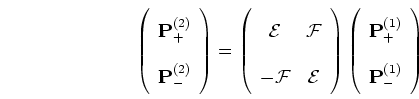(6.24)

where
is an
matrix given by
(6.25)

and
is anmatrix given by
(6.26)

Notice that if there is no backward going wave in section 2, then
, and from equation (6.24),
which agrees with the results of section 6.3.

Each of the elements in equation (6.24) is frequency dependent so we must compute the scattering matrix for each frequency in the Fourier transform of the pressure signals and perform the matrix multiplication in the frequency domain. This method means a significant increase in the computational load. Only a small number of modes could be added for this method of bore reconstruction to be feasible.

In addition to scattering across discontinuities, scattering along cylindrical sections is also necessary. We will therefore require multimodal versions of equations (6.18) and (6.19) which give the pressure value at surface 3 from the pressure value at surface 2 in figure 5.1. The modes propagate independently between these surfaces because the cross-section remains constant. For a given frequency of sound, the wavelength along the axis of the duct depends on the mode under consideration, with higher order modes having longer wavelengths untill at high enough mode numbers the waves are exponentially damped. This means the pressure is not just delayed by travel along the pipe, it also experiences dispersion.

The dispersion is described in the time domain by Morse and Ingard  p.498. Firstly, below cut-off the
modes are exponentially damped meaning that the low frequencies are filtered out. Above cut-off, the phase velocity is larger than
and the group velocity is smaller than, with both converging onin the high frequency limit. The time domain impulse transfer function for a higher mode pressure distribution is calculated by Fourier transform to give an impulse travelling at the speed of soundwith a wake trailing behind. Physically this is realistic since an impulse contains all frequency components, with the very high frequencies responsible for the sharp impulse travelling with a group velocity ofand the lower frequency components following behind.

Using this impulse transfer function for time domain convolution with the forward and backward going pressure vectors is a possible solution for scattering down a cylindrical section. Alternatively, frequency domain multiplication can be used. The pressure amplitude of theth higher mode in a uniform pipe is described in equation (2.33). Projecting the forward going part gives
(6.27)

and the backward going part gives(6.28)

Back to Kemp Acoustics HomeNext: Iteration method with multimodal Up: Issues in multimodal bore Previous: Issues in multimodal bore   Contents
Jonathan Kemp 2003-03-24